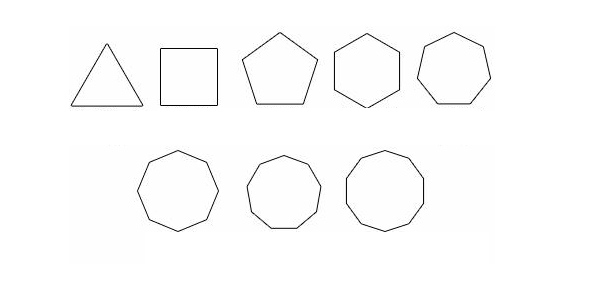# Polygons

10 Questions | Total Attempts: 92SettingsClassifying polygons by their sides

Related Topics
• 1.
A polygon with 3 sides is called?
• 2.
A seven sided polygon is called a nonagon.
• A.

True

• B.

False

• 3.
A polygon with 8 sides is called?
• 4.
A trapezoid is a type of quadrilateral?
• A.

True

• B.

False

• 5.
Classify the polygon that has 6 sides.
• A.

Pentagon

• B.

Decagon

• C.

Hexagon

• 6.
Which of the following polygons is classified as a quadrilateral?
• A.

Parallelogram

• B.

Triangle

• C.

Octagon

• 7.
A polygon with 5 sides is called?
• 8.
Define congruent
• A.

Figures are same size

• B.

Figures are same size and shape

• C.

Figures are similar

• 9.
How many lines of symmetry does a regular octagon have?
• 10.
How many lines of symmetry does a square have?
• A.

2

• B.

4

• C.

0